## Example Questions

← Previous 1 3

### Example Question #91 : Percentage

An organic farmer has had a stall at the market for three years.  The first year she sold 500 pounds of tomatoes.  The second year she sold 650 pounds of tomatoes.  The third year she sold 750 pounds of tomatoes.  What is the percentage increase in sales from year 1 to 2 and year 2 to 3? Round to one decimal point.

20.0% / 15.4%

30.0% / 15.4%

30.0% / 20.0%

20.0% / 23.1%

50.0% / 20%

30.0% / 15.4%

Explanation:

Year 2 Percentage increase =

(Year 2 – Year 1) / Year 1 =

(650 – 500) / 500 = 150 / 500 = .3 = 30.0%

Year 3 Percentage increase =

(Year 3 – Year 2) / Year 2 =

(750 – 650) / 650 = 100 / 650 = .154 = 15.4%

### Example Question #91 : Percentage

If there are 36 students in a class and the teacher wants the top 25% of the students to move up to the advanced level course. How many students will remain in the class?

26

28

30

27

29

27

Explanation:

The key here is to find 25% of the total class (36), and subtract that value from the total (36). You can also look at the problem from reversely and find how many students are in the remaining 75% of the total 36. Remember to change the percent to a decimal for easy calculation. 36- 0.25(36)= 27 or 0.75(36)=27

### Example Question #1 : How To Find Percentage

What percent of 240 is 12?

5%

7.5%

10%

12%

25%

5%

Explanation:

"Is" means an equal sign and "of" means multiplication.

0.01z * 240 = 12 and solving for z = 0.05 or 5%

### Example Question #1 : Other Percentage

A person sleeps six hours each day.  Monday through Friday, he spends 75% of his waking day in work-related activities (commuting, working, etc).  Monday through Sunday, he spends 10% of his waking hours attending to vital needs (cleaning, eating, etc).  If this person does not work on Saturday or Sunday, how much free time (to the closest minute) does he have every week?

42 hours, 41 minutes

41 hours, 42 minutes

45 hours, 54 minutes

54 hours, 54 minutes

65 hours, 2 minutes

45 hours, 54 minutes

Explanation:

Let's consider our data in a summary fashion:

The day begins with 24 hours.  After sleeping 6 of those, the person has 18 remaining hours.  Of those, 10% are given to vital tasks every day.  That means that he uses 18 * 0.1 or 1.8 hours each day for these tasks.  Therefore, he has 16.2 hours remaining.  This will be free time on Saturday and Sunday.

During the week, however, he uses 75% of his waking time in work activities.  This means that he spends 18 * 0.75 or 13.5 hours.  He therefore has 16.2 - 13.5 or 2.7 hours.

Now, to figure out the total amount for the week, he has 16.2 for the two weekend days and 2.7 for the five week days, or: 16.2 * 2 + 2.7 * 5 = 32.4 + 13.5 = 45.9hours.  This is equal to 45 hours and 0.9 * 60, or 54, minutes.

### Example Question #5 : How To Find Percentage

A bag contains three types of marbles: red, green, and yellow. 75% of the marbles are green or yellow. Which of the following values is valid in this case?

15 Yellow, 32 Green, 10 Red

3 Yellow, 1 Green, 4 Red

12 Yellow, 32 Green, 2 Red

13 Yellow, 32 Green, 15 Red

13 Yellow, 32 Green, 15 Red

Explanation:

We have to consider each case:

15 Yellow, 32 Green, 10 Red

Total: 15 + 32 + 10 = 57; Yellow and Green: 15 + 32 = 47.

Percentage that are Yellow and Green = 47/57, or approximately 82.47%

12 Yellow, 32 Green, 2 Red

Total: 12 + 32 + 2 = 46; Yellow and Green: 12 + 32 = 44.

Percentage that are Yellow and Green = 44/46 or approximately 95.65%

3 Yellow, 1 Green, 4 Red

Total: 3 + 1 + 4 = 8; Yellow and Green: 3 + 1 = 4.  Percentage that are Yellow and Green = 4/8 or 50%.

13 Yellow, 32 Green, 15 Red

Total: 13 + 32 + 15 = 60; Yellow and Green: 13 + 32 = 45

Percentage that are Yellow and Green = 45 / 60 or 75%. This is the answer.

### Example Question #1 : How To Find Percentage

On average, professional poker player Ben wins 6 out of 10 games. If a win brings in $75, how many games should he play to cover his rent of$625?

5

23

19

30

14

14

Explanation:

Put into an equation where x is the number of games, 0.6 * x * 75 = 625. X = 13.9, the answer is 14.

### Example Question #7 : How To Find Percentage

What percent of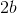is?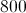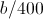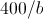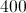Explanation:

Set up a ratio where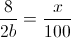and solve forthrough cross multiplication.

### Example Question #8 : How To Find Percentage

In a jar of 240 coins, 20 percent are nickels and 5 percent are dimes. How many coins are not nickels or dimes?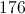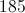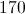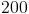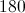Explanation:

75% of the coins are not nickels or dimes. This means thatcoins are not nickels or dimes.

### Example Question #9 : How To Find Percentage

What number is 125% of 120?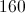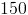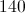Explanation:

Verbal cues include "of" and "is."  Of means multiplication and is means equals

So the equation to solve becomes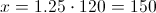### Example Question #10 : How To Find Percentage

What percent of 250 is 20?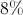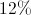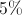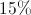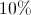Explanation:

Verbal cues include "of" and "is."  Of means multiplication and is means equals

The equation to solve becomes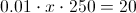so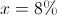← Previous 1 3

### All SAT Math Resources